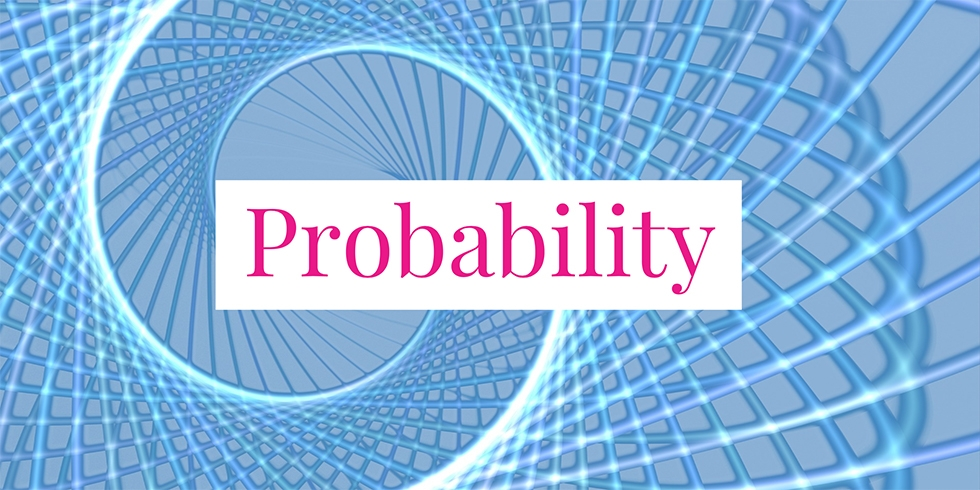# Lower and Upper Bounds of the Probability of the Intersection of Two Events## Problem 741

Let $A, B$ be events with probabilities $P(A)=2/5$, $P(B)=5/6$, respectively. Find the best lower and upper bound of the probability $P(A \cap B)$ of the intersection $A \cap B$. Namely, find real numbers $a, b$ such that
$a \leq P(A \cap B) \leq b$ and $P(A \cap B)$ could take any values between $a$ and $b$.Add to solve later

## Solution.

For the lower bound, we use the basic equality of the probability theory that
$P(A \cap B) = P(A) + P(B) – P(A \cup B).$ Note also that we always have the inequality $P(A \cup B) \leq 1$. Altogether we obtain the following inequality.
\begin{align*}
P(A \cap B) &= P(A) + P(B) – P(A \cup B)\\
&\geq P(A) + P(B) – 1\5pt] &= \frac{2}{5} + \frac{5}{6} – 1\\[5pt] &= \frac{12 + 25 -30}{30}\\[5pt] &= \frac{7}{30}. \end{align*} This gives the lower bound a = 7/30. Note that P(A \cap B) could take this lower bound when P(A \cup B) = 1 and this happens if A\cup B is the whole sample space. Next, we’ll obtain the upper bound. As the intersection A \cap B is contained in the set A and in the set B, we have \begin{align*} P(A \cap B) &\leq \min(P(A), P(B))\\ &= \min \left(\frac{2}{5}, \frac{5}{6}\right)\\ & = \frac{2}{5}. \end{align*} This yields the upper bound b = 2/5. The probability P(A \cap B) could take this upper bound when A \cap B = A (this happens when A \subset B). In conclusion, we obtain the following bounds \[\frac{7}{30} \leq P(A \cap B) \leq \frac{2}{5}.

We remark that as a probability we clearly have bounds $0 \leq P(A \cap B) \leq 1$. However, these bounds are not optimal in a sense that $P(A \cap B)$ never takes values less than $7/30$ or above $2/5$ as we determined above.Add to solve later

### More from my site

#### You may also like...

This site uses Akismet to reduce spam. Learn how your comment data is processed.

###### More in Probability##### Find the Conditional Probability About Math Exam Experiment

A researcher conducted the following experiment. Students were grouped into two groups. The students in the first group had more...

Close